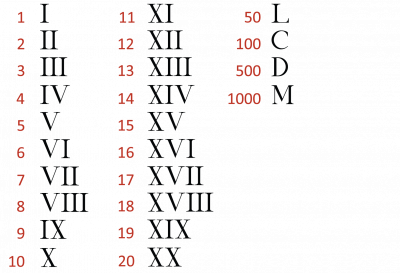The Romans used capital letters to write their numbers. Roman numbers are called numerals.If you put a letter after a larger one it means you add it.

Here is an example:

LII = 52

This means 50 (L) + 2 (II) = 52

50 + 2 = 52

If you put a letter before a larger one it means you take it away.

Here is an example:

XC = 90

This means you take 10 (X) away from 100 (C)

100 - 10 = 90

Now that you have read some examples of Roman numerals, let's start the activities.